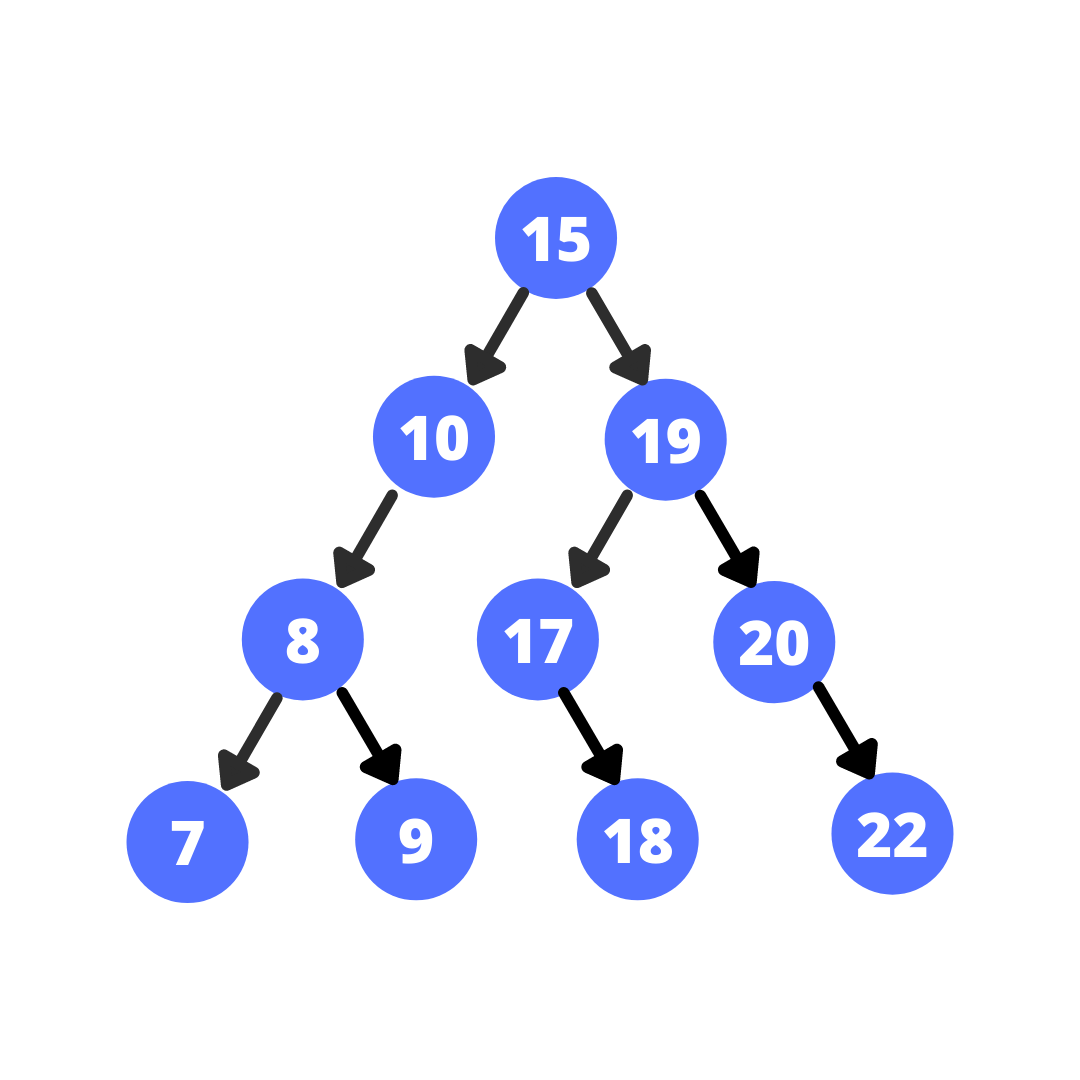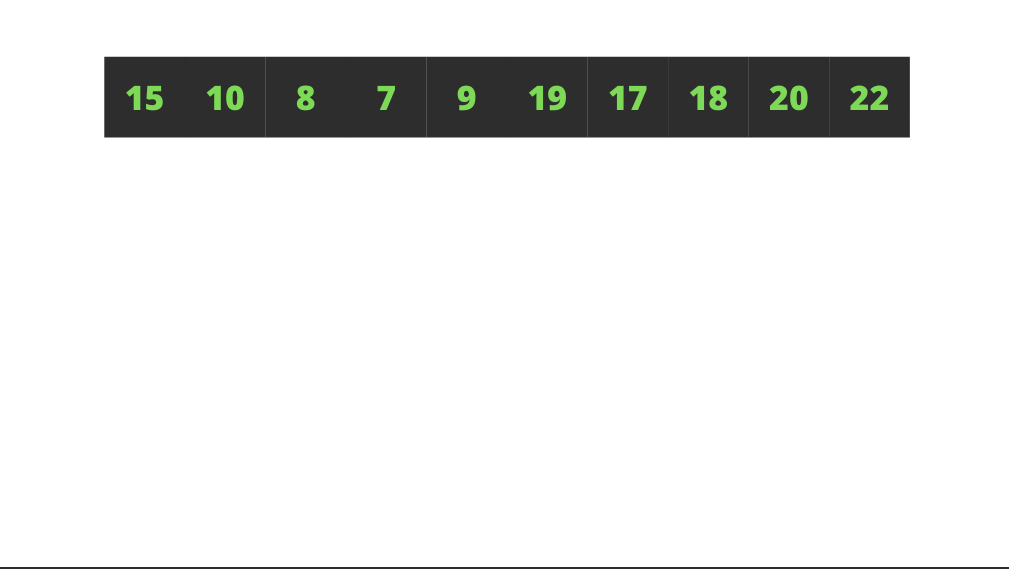# Binary Search Trees (BST) - II

##### Constructing using the given preorder traversalBatoi Research Group Jun 18, 2021

This article will show how to construct a binary search tree if a pre-order traversal is given. Let’s say we have a pre-order traversal as [15,10,8,7,9,19,17,18,20,22], and we have to construct the following Binary Search Tree.### 1. Brute Force/Naive approach

In the pre-order traversal, the first node that we see is the root node, so we can easily say that root = pre the rest of the values either come in the left subtree or the right subtree.

Since the nodes of the left subtree are smaller than the root, we can traverse the list until we find the element that is greater than the root. All the elements before it (except root) will be in the left subtree, and the remaining will be in the right subtree. From here, we can use the same idea recursively to construct the entire BST.CODE:



# include

using namespace std;

class TreeNode{ public: int val; TreeNode left, right; TreeNode(int data){ val = data; left = right = NULL; } };

void inorder(TreeNode root){ if(!root) return; inorder(root->left); coutleft = createBST(pre,low+1,idx-1); root->right = createBST(pre,idx,high); return root; } int main() { int pre[] = {15,10,8,7,9,19,17,18,20,22}; int n = sizeof(pre)/sizeof(pre); TreeNode root; root = createBST(pre,0,n-1); inorder(root); return 0; } 

Time complexity: O(n2)

Space complexity: O(n) due to recursion

### 2.Optimal Approach (Recursive)

Similar to what we did while verifying that a Tree is a BST, we shall have a range for each node. A node that comes in the range forms a root of the tree.

Initially, the range is [-inf, inf]. The first node will be in the range, so it will become the root of the tree. The range for the left subtree will be [-inf, root->data] and the range for the right subtree will be [root->data, inf]. From here, we will call the same method for the left and right subtree recursively to construct the entire BST.

CODE:



# include

using namespace std;

class TreeNode{ public: int val; TreeNode left, right; TreeNode(int data){ val = data; left = right = NULL; } }; int size; int idx;

void inorder(TreeNode root){ if(!root) return; inorder(root->left); cout min && pre[idx] < max) { root = new TreeNode(pre[idx]); idx++; root->left = createBST(pre,min,root->val); root->right = createBST(pre,root->val,max); } return root; } int main() { int pre[] = {15,10,8,7,9,19,17,18,20,22}; size = sizeof(pre)/sizeof(pre); idx = 0; TreeNode root; root = createBST(pre,INT_MIN,INT_MAX); inorder(root); return 0; } 

Time complexity: O(n)

Space complexity: O(n) due to recursion

### 3.Optimal Approach (Iterative)

Here we will see a different O(n) approach using stack instead of recursion.

1. Create an empty stack.

2. Push the first element to the stack.

3. Keep popping the elements of the stack while the stack is not empty and the next element in the pre-order list is greater than the top value of the stack. Also, store the value being popped in a temp variable which is initially NULL.

4. If the temp is null (that is current element was smaller than the stack’s top), make the current element the left of the child of the stack’s top. Push the new element to the stack.

5. Else, make the current element the right child of temp and push the new node to stack. Repeat steps 3,4,5 until there is no element left in the pre-order list. Return root.

CODE:



# include

using namespace std class TreeNode{ public: int val; TreeNode left, right; TreeNode(int data){ val = data; left = right = NULL; } };

void inorder(TreeNode root){ if(!root) return; inorder(root->left); coutleft = new TreeNode(pre[i]); s.push(temp->left); } else{ temp->right = new TreeNode(pre[i]); s.push(temp->right); } } return root; } int main() { int pre[] = {15,10,8,7,9,19,17,18,20,22}; size = sizeof(pre)/sizeof(pre); TreeNode root; root = createBST(pre); inorder(root); return 0; } 

Time complexity: O(n)

Space complexity: O(n) due to the use of stack

### Need our assistance? We are available with you.